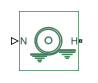# Rolling Resistance

Model rolling resistance

•Libraries:
Simscape / Driveline / Tires & Vehicles / Tire Subcomponents

## Description

The Rolling Resistance block represents the resistance force that acts on the wheel hub due to the rolling resistance at the road-wheel contact surface. The block can use a constant resistance coefficient of the pressure and velocity dependence of the SAE J2452 standard, or the Magic Formula. The resistance force is zero when the normal force acting at the wheel-road surface is less than or equal to zero.

### Constant Resistance Coefficient Model

When you set Resistance model to ```Constant coefficient```, the rolling resistance is directly proportional to the resistance coefficient F = , where:

• F is the rolling resistance force.

• N is the normal force.

• μ is the rolling resistance coefficient.

The rolling resistance coefficient has a hyperbolic form that eliminates discontinuity at vhub = 0

`$\mu ={\mu }_{0}\cdot \mathrm{tanh}\left(\frac{4\cdot {v}_{hub}}{{v}_{threshold}}\right),$`

where:

• μ0 is the asymptotic rolling resistance coefficient.

• vhub is the hub velocity.

• vthreshold is the threshold velocity.

### Pressure and Velocity Dependent Model

When you set Resistance model to ```Pressure and velocity dependent (SAE J2542)```, the block uses the formula:

`$F={\left(\frac{P}{{P}_{0}}\right)}^{\alpha }{\left(\frac{N}{{N}_{0}}\right)}^{\beta }{N}_{0}\cdot \left(A+B|{v}_{hub}|+C{v}_{hub}{}^{2}\right),$`

where:

• P is the tire pressure.

• vhub is the hub velocity.

• α, β, A, B, and C are the approximating coefficients.

• P0 is 1 Pascal (Pa).

• N0 is 1 Newton (N).

In this equation, the parameters P0 and N0 remove the physical units from each exponential expression base.

When you set Resistance model to ```Pressure and velocity dependent (Magic Formula)```, the block uses the Magic Formula to calculate rolling resistance as

`$F={F}_{Z0}{\lambda }_{My}\left({q}_{sy1}+{q}_{sy2}\frac{{F}_{X,Tread}}{{F}_{Z0}}+{q}_{sy3}|\frac{{V}_{H}}{{V}_{0}}|+{q}_{sy4}{\left(\frac{{V}_{H}}{{V}_{0}}\right)}^{4}\right){\left(\frac{{F}_{Z}}{{F}_{Z0}}\right)}^{{q}_{sy7}}{\left(\frac{{p}_{i}}{{p}_{i0}}\right)}^{{q}_{sy8}},$`

where:

• FZ0 is the Tire nominal vertical load, FNOMIN parameter.

• λMy is the Scale factor of rolling resistance, LMY parameter.

• qsy1,...,qsy8 are the elements of the Q-coefficient parameters [qsy1 qsy2 qsy3 qsy4 qsy5 qsy6 qsy7 qsy8] parameter.

• V0 is the Hub nominal longitudinal speed, LONGVL parameter.

• pi is the Tire pressure parameter.

• pi0 is the Tire nominal pressure, NOMPRES parameter.

• VH is the wheel hub longitudinal velocity.

• FZ is the vertical load on the tire.

Use port T to input the friction force from the road on the tire.

## Ports

### Input

expand all

Physical signal input port associated with the normal force, in N. The positive force direction is down.

Physical signal input port associated with the tread friction force, in N. The positive force direction is forwards.

#### Dependencies

To enable this port, set Resistance model to ```Pressure and velocity dependent (Magic Formula)```.

### Conserving

expand all

Mechanical translational conserving port associated with the wheel hub, in m/s.

## Parameters

expand all

Method to compute the rolling resistance on a wheel hub. The parameter has three options:

• `Constant coefficient`

• ```Pressure and velocity dependent (SAE J2542)```

• ```Pressure and velocity dependent (Magic Formula)```

Constant coefficient value to compute the rolling resistance.

#### Dependencies

To enable this parameter, set Resistance model to `Constant coefficient`.

Constant inflation pressure of the rolling tire.

#### Dependencies

To enable this parameter, set Resistance model to ```Pressure and velocity dependent (SAE J2542)``` or ```Pressure and velocity dependent (Magic Formula)```.

SAE J2452 coefficient for the pressure and velocity parameterization. You determine this value using empirical data in accordance with SAE J2452.

#### Dependencies

To enable this parameter, set Resistance model to ```Pressure and velocity dependent (SAE J2542)```.

SAE J2452 coefficient for the pressure and velocity parameterization. You determine this value using empirical data in accordance with SAE J2452.

#### Dependencies

To enable this parameter, set Resistance model to ```Pressure and velocity dependent (SAE J2542)```.

SAE J2452 coefficient for the pressure and velocity parameterization. You determine this value using empirical data in accordance with SAE J2452.

#### Dependencies

To enable this parameter, set Resistance model to ```Pressure and velocity dependent (SAE J2542)```.

SAE J2452 coefficient for the pressure and velocity parameterization. You determine this value using empirical data in accordance with SAE J2452.

#### Dependencies

To enable this parameter, set Resistance model to ```Pressure and velocity dependent (SAE J2542)```.

SAE J2452 coefficient for the pressure and velocity parameterization. You determine this value using empirical data in accordance with SAE J2452.

#### Dependencies

To enable this parameter, set Resistance model to ```Pressure and velocity dependent (SAE J2542)```.

Nominal tire pressure. `NOMPRES` it the TIR file identifier.

#### Dependencies

To enable this parameter, set Resistance model to ```Pressure and velocity dependent (Magic Formula)```.

Nominal normal force, Fz0, on tire. `FNOMIN` is the TIR file identifier.

#### Dependencies

To enable this parameter, set Resistance model to ```Pressure and velocity dependent (Magic Formula)```.

Nominal hub longitudinal speed. `LONGVL` is the TIR file identifier.

#### Dependencies

To enable this parameter, set Resistance model to ```Pressure and velocity dependent (Magic Formula)```.

Magic Formula Q coefficients. `qsy1`,…, `qsy8` correspond to the TIR file identifiers.

#### Dependencies

To enable this parameter, set Resistance model to ```Pressure and velocity dependent (Magic Formula)```.

Scale factor of the rolling resistance, λMY. `LMY` is the TIR file identifier.

#### Dependencies

To enable this parameter, set Resistance model to ```Pressure and velocity dependent (Magic Formula)```.

Minimum velocity that attains the maximum amount of rolling resistance.

## Version History

Introduced in R2012a

expand all